# How to Calculate and Solve for Glass Gas Solubility in a Fluid | The Calculator Encyclopedia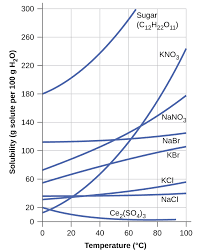The image above represents glass gas solubility.

To compute for the glass gas solubility, four essential parameters are needed and these parameters are API Gravity (°API), Temperature (°Rankine) (T), Mean Bubble Point (Pb*) and Gas Gravity at Actual Separator Psep and Tsepg).

The formula for calculating the glass gas solubility:

γgs = γg[((°API)0.989 / (T – 460)0.172)Pb*]1.2255

Where:

γgs = Glass Gas Solubility
°API = API Gravity
T = Temperature (°Rankine)
Pb* = Mean Bubble Point
γg = Gas Gravity at Actual Separator Psep and Tsep

Let’s solve an example;
Find the glass gas solubility when the API Gravity is 15, the temperature is 30, the mean bubble point is 40 and the gas gravity at actual separator is 54.

This implies that;

°API = API Gravity = 15
T = Temperature (°Rankine) = 30
Pb* = Mean Bubble Point = 40
γg = Gas Gravity at Actual Separator Psep and Tsep = 54

γgs = γg[((°API)0.989 / (T – 460)0.172)Pb*]1.2255
γgs = 54[((15)0.989 / (30 – 460)0.172)40]1.2255
γgs = 54[((15)0.989 / (-430)0.172)40]1.2255
γgs = 54[((15)0.989 / (NaN))40]1.2255
γgs = 54[(14.5 / NaN)40]1.2255
γgs = 54[(NaN)40]1.2255
γgs = 54[NaN]1.2255
γgs = 54[NaN]
γgs = NaN

Therefore, the glass gas solubility is NaN.

Nickzom Calculator – The Calculator Encyclopedia is capable of calculating the glass gas solubility.

To get the answer and workings of the glass gas solubility using the Nickzom Calculator – The Calculator Encyclopedia. First, you need to obtain the app.

You can get this app via any of these means:

To get access to the professional version via web, you need to register and subscribe for NGN 1,500 per annum to have utter access to all functionalities.
You can also try the demo version via https://www.nickzom.org/calculator

Android (Paid) – https://play.google.com/store/apps/details?id=org.nickzom.nickzomcalculator
Android (Free) – https://play.google.com/store/apps/details?id=com.nickzom.nickzomcalculator
Apple (Paid) – https://itunes.apple.com/us/app/nickzom-calculator/id1331162702?mt=8
Once, you have obtained the calculator encyclopedia app, proceed to the Calculator Map, then click on Petroleum under EngineeringNow, Click on Fluid Properties under Petroleum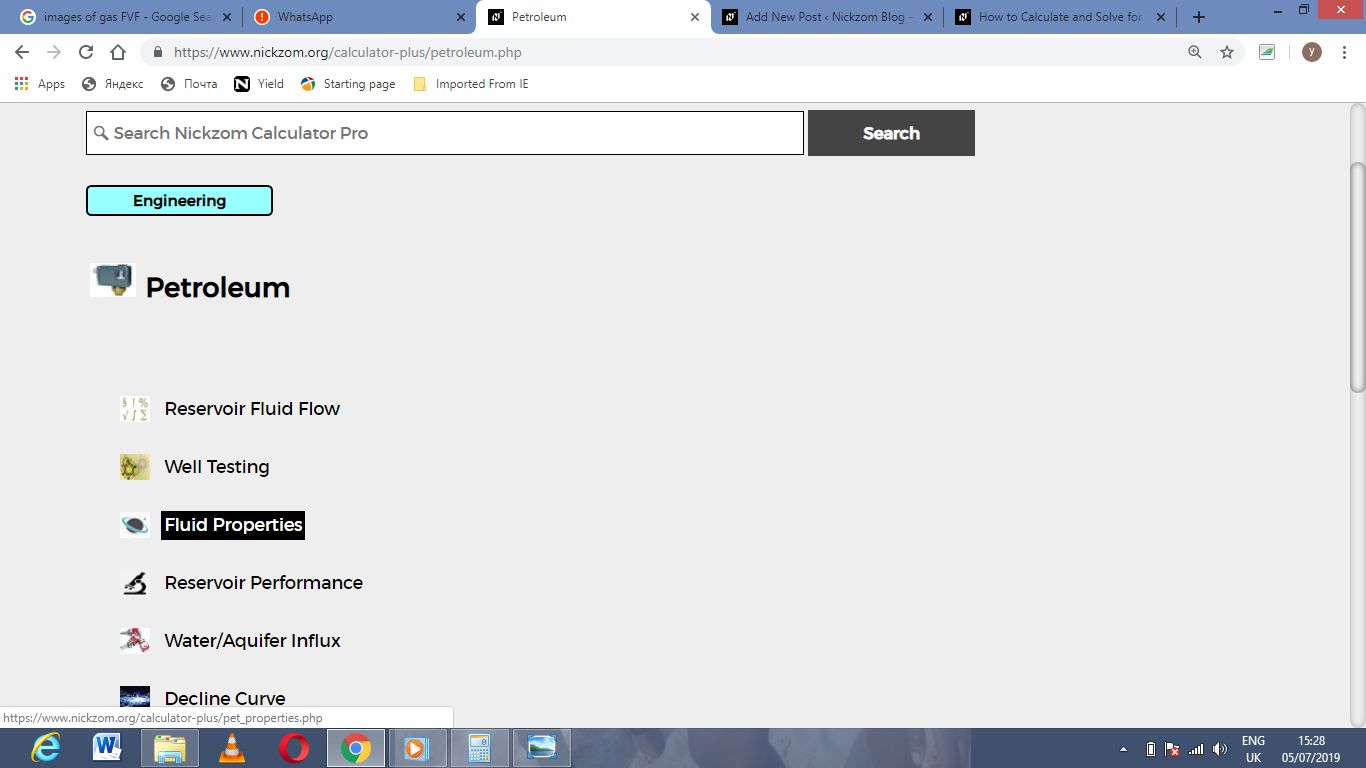Now, Click on Glass Gas Solubility under Fluid Properties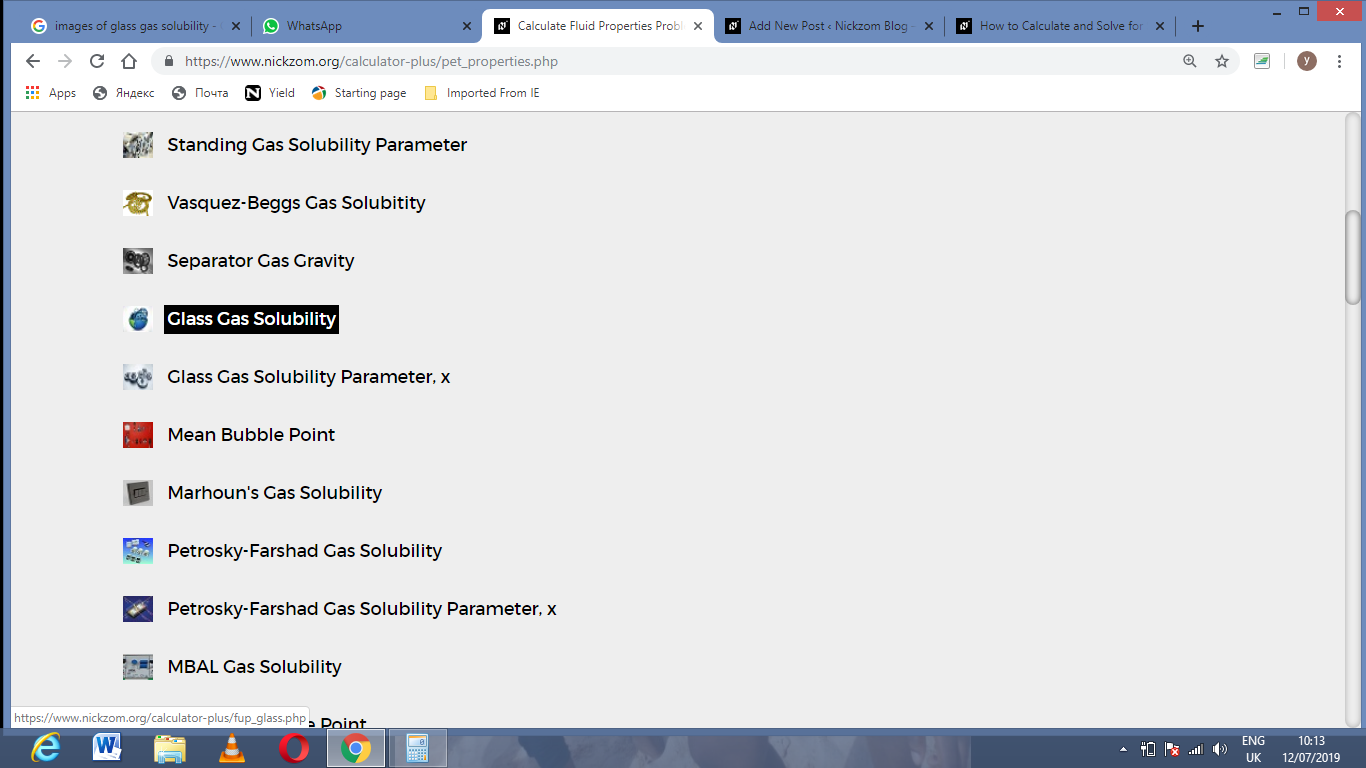The screenshot below displays the page or activity to enter your values, to get the answer for the glass gas solubility according to the respective parameters which are the API Gravity (°API), Temperature (°Rankine) (T), Mean Bubble Point (Pb*) and Gas Gravity at Actual Separator Psep and Tsepg).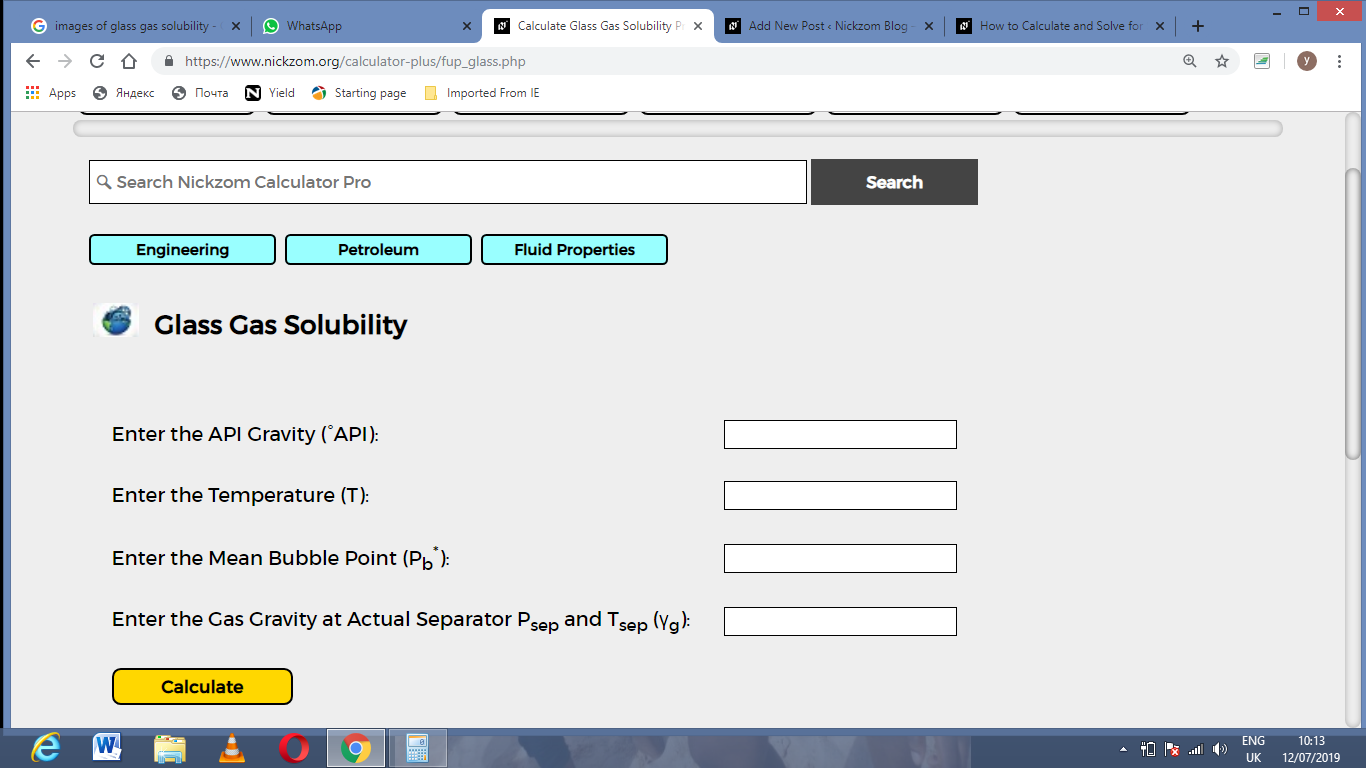Now, enter the values appropriately and accordingly for the parameters as required by the API Gravity (°API) is 15, Temperature (°Rankine) (T) is 30, Mean Bubble Point (Pb*) is 40 and Gas Gravity at Actual Separator Psep and Tsepg) is 54.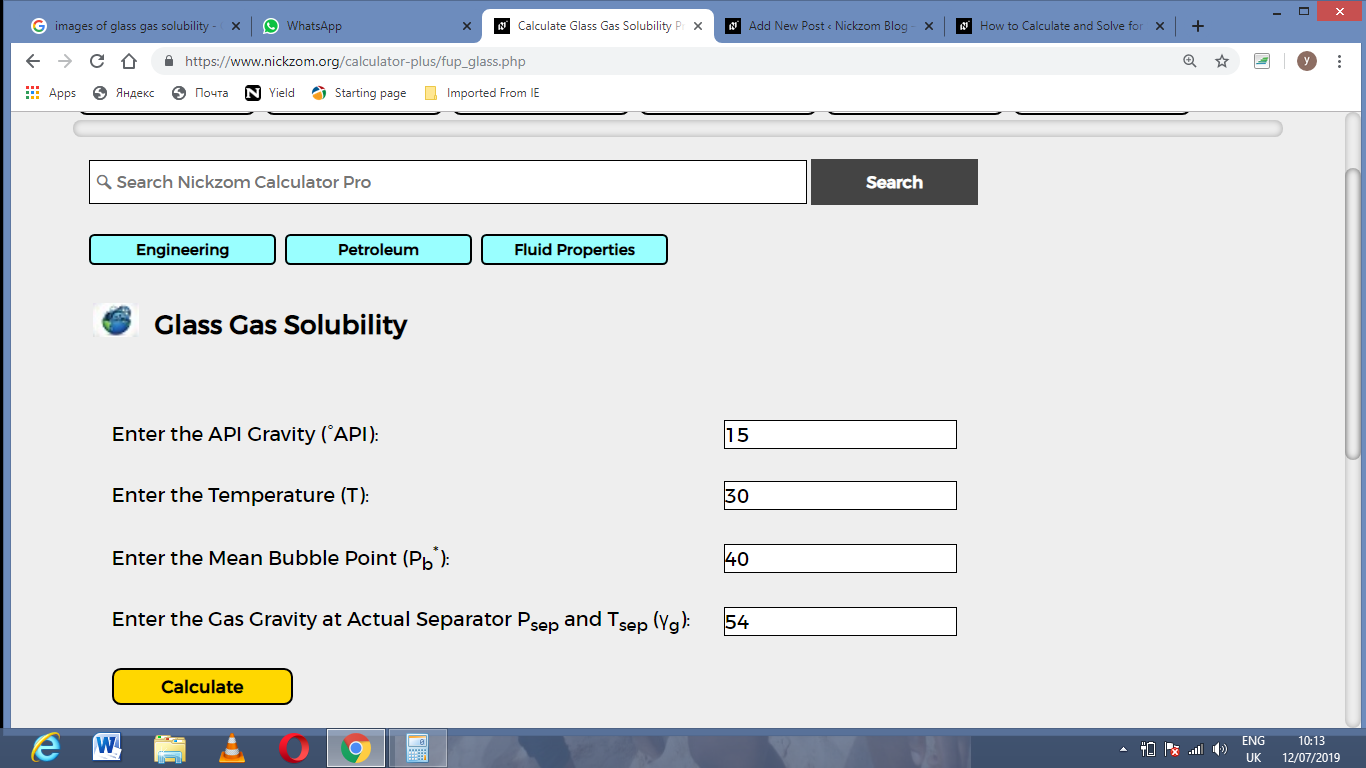Finally, Click on Calculate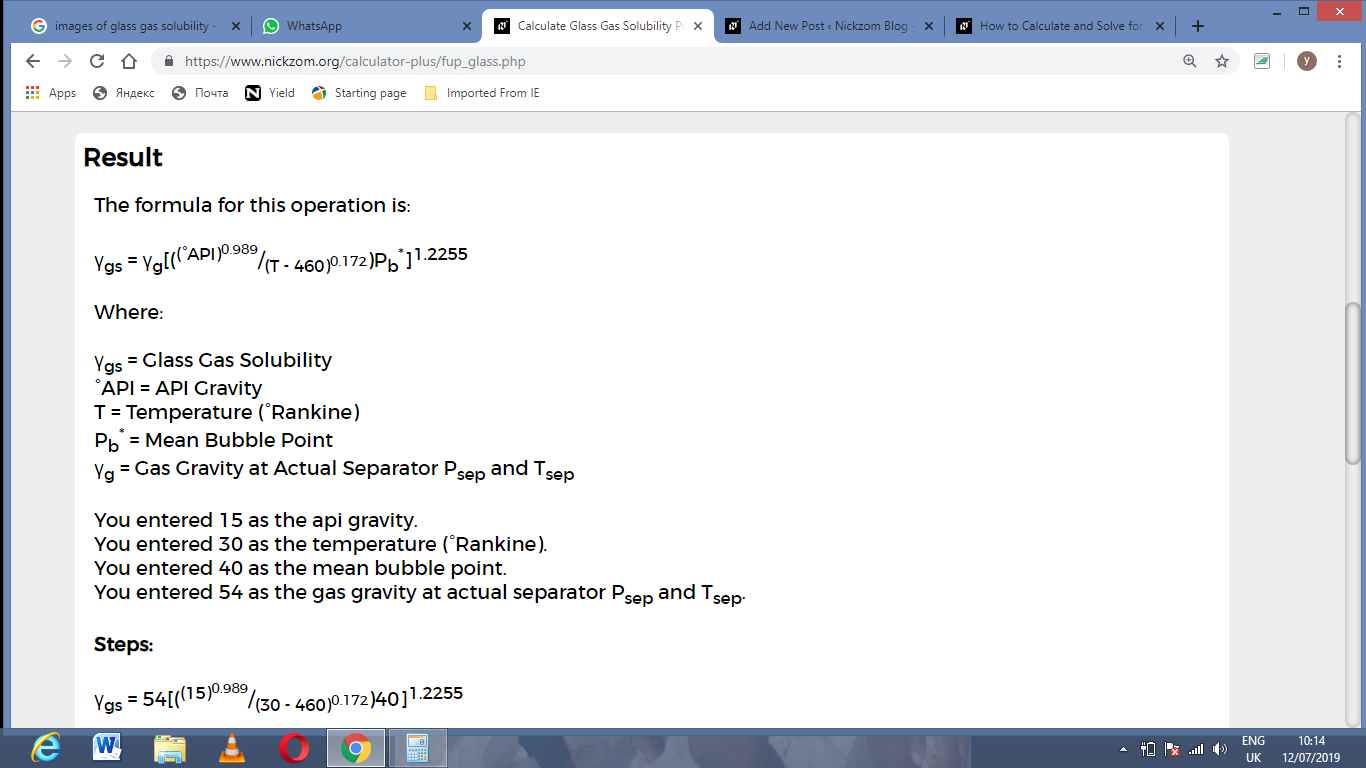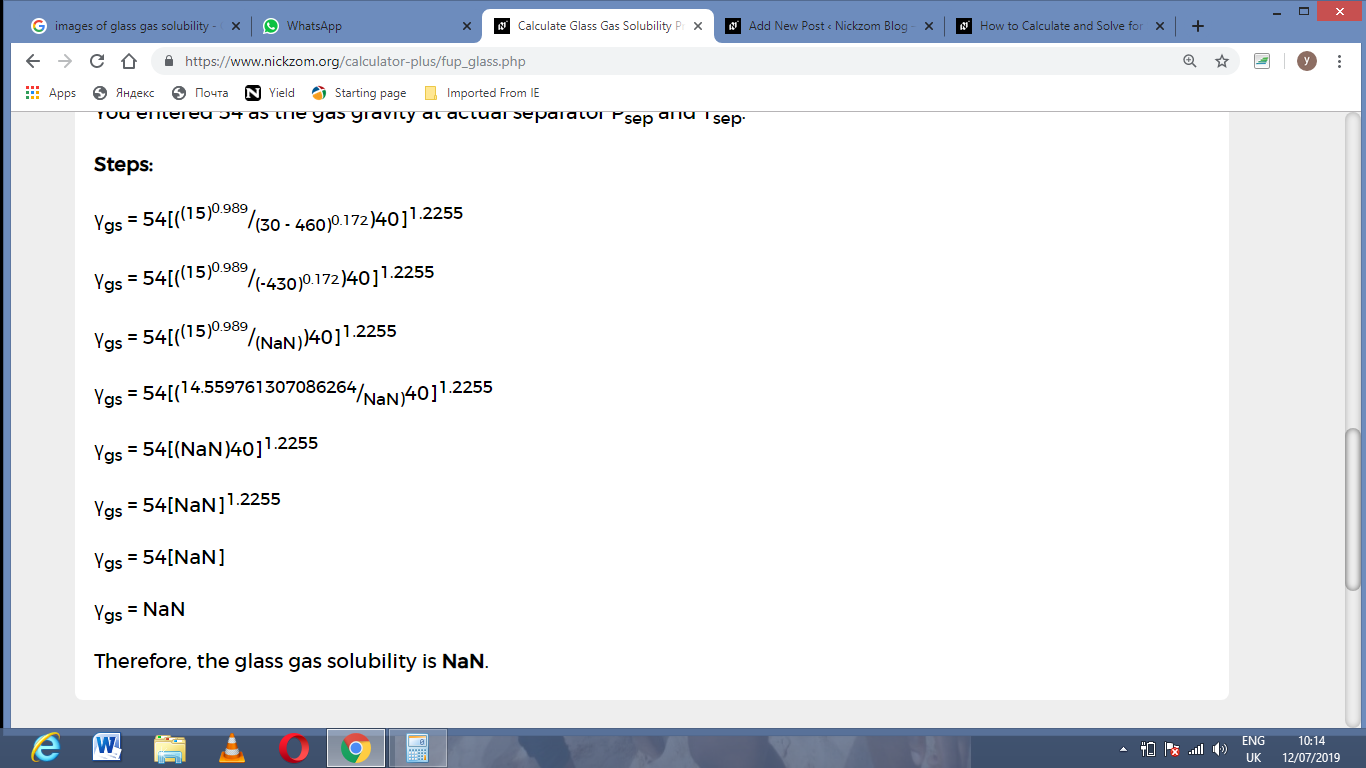As you can see from the screenshot above, Nickzom Calculator– The Calculator Encyclopedia solves for the glass gas solubility and presents the formula, workings and steps too.